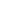#Introduction to Probability and Statistics is a Course

## Introduction to Probability and Statistics

Time limit: 90 days

### Full course description

Uncertainty plays a major role in the business world. You may not be able to fully predict the outcome of a decision, but you can use mathematical tools to help you make informed decisions and manage risks. This course will introduce you to the concepts of probability and statistics. You will learn how to calculate probabilities in a variety of situations. You will also explore simple ways to organize and analyze data by learning about different graphical displays and basic statistical terminology.

Learning Outcomes:

Use the properties of sets in real-world situations

Count the number of possible outcomes in a variety of situations, such as the number of permutations and combinations for a set

Calculate the probability of simple events occurring

Calculate probabilities in a variety of situations, including conditional events, dependent events, and independent events

Differentiate between qualitative and quantitative data

Interpret data from graphical displays including histograms, pie charts, and bar charts

Explain the real-world meaning of descriptive statistics, such as measures of central tendency and spread

Explain the real-world meaning of percentiles for a data set

Describe the role outliers play on the distribution of a data set

Credits:

IACET CEUs: 0.6 (Contact Hours: 6 hours)

Estimated time to complete: 6 hours